Name:    Geometry - Chapter 1 Practice Test

1.

Name three points that are collinear.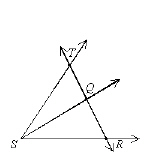a. points T, Q, and R c. points S, Q, and R b. points T, Q, and S d. points T, S, and R

2.

Draw a labeled diagram for an angle.
 a.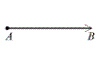c.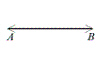b.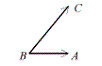d.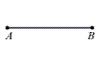3.

The notation for the length of the segment between P and Q is _______.
 a.b.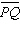c.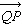d. PQ

4.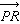is represented by which sketch?
 a.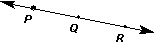c.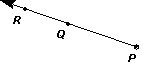b.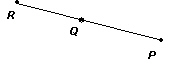d.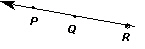5.

If AB = 17 and AC = 32, find the length of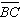.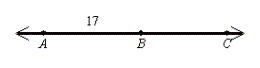a. 17 b. 5 c. 49 d. 15

6.

Find the midpoint of the segment with endpoints (–2, 4) and (–4, 3).
 a.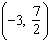b.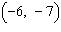c.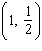d.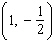7.

The diagonals of parallelogram ABCD have a common midpoint.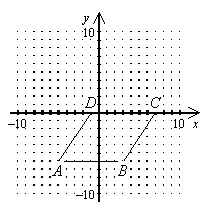Which of the following is the midpoint of the diagonals of ABCD?
 a.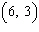b.c.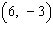d.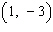8.

Which angle measures approximately 112°?
 a.c.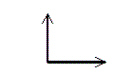b.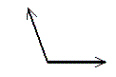d.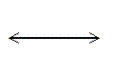9.

If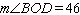° and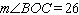°, then what is the measure of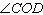?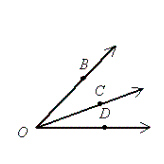a. 25° b. 20° c. 17° d. 18°

10.

If angle TOS is acute and angle TOR is right, then angle ROS is what kind of angle?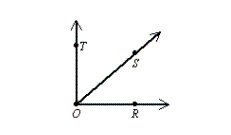a. obtuse b. right c. straight d. acute

11.

If an obtuse angle is bisected, the resulting angles are _________.
 a. always acute c. never congruent b. right angles d. always obtuse

12.

Which does not name the angle below?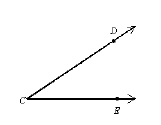a.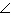DCE b.CDE c.ECD d.C

13.

The nonshared sides of two adjacent angles form a pair of opposite rays. The angles are _________.
 a. acute b. complementary c. a linear pair d. vertical angles

14.

Name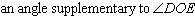.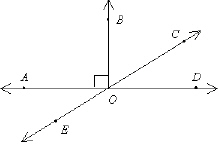a.c.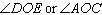b.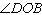d.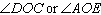15.

Which is not a possible value for y in the figure below?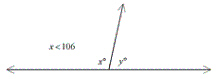a. 151 b. 79 c. 66 d. 106

Complete the conditional statement to make a true statement.

16.

If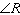and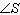are complementary and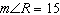°, then
 a.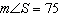° b.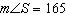° c.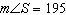° d.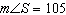°

17.

Find the circumference of the circle. Use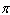= 3.14.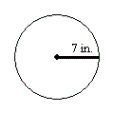a. 87.92 in. b. 153.86 in. c. 43.96 in. d. 7.72 in.

18.

A wooden fence is to be built around a 28 m-by-76 m lot. How many meters of fencing will be needed? If the wood for the fence costs \$28.00 per meter, what will the wood for the fence cost?
 a. 2128 m, \$5,824.00 c. 2128 m, \$59,584.00 b. 208 m, \$59,584.00 d. 208 m, \$5,824.00